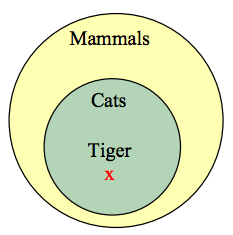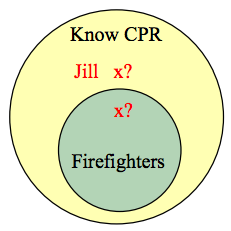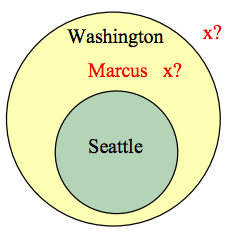## Truth Tables

Because complex Boolean statements can get tricky to think about, we can create a truth table to keep track of what truth values for the simple statements make the complex statement true and false

### Truth Table

A table showing what the resulting truth value of a complex statement is for all the possible truth values for the simple statements.

### Example 1

Suppose you’re picking out a new couch, and your significant other says “get a sectional or something with a chaise.”

This is a complex statement made of two simpler conditions: “is a sectional,” and “has a chaise.” For simplicity, let’s use S to designate “is a sectional,” and C to designate “has a chaise.” The condition S is true if the couch is a sectional.

A truth table for this would look like this:

S C S or C
T T T
T F T
F T T
F F F

In the table, T is used for true, and F for false. In the first row, if S is true and C is also true, then the complex statement “S or C” is true. This would be a sectional that also has a chaise, which meets our desire.

Remember also that or in logic is not exclusive; if the couch has both features, it does meet the condition.

To shorthand our notation further, we’re going to introduce some symbols that are commonly used for and, or, and not.

### Symbols

The symbol ⋀ is used for and: A and B is notated AB.

The symbol ⋁ is used for or: A or B is notated A ⋁ B

The symbol ~ is used for not: not A is notated ~A

You can remember the first two symbols by relating them to the shapes for the union and intersection. A B would be the elements that exist in both sets, in A ⋂ B. Likewise, A B would be the elements that exist in either set, in A ⋃ B.

In the previous example, the truth table was really just summarizing what we already know about how the or statement work. The truth tables for the basic and, or, and not statements are shown below.

### Basic Truth Tables

A B A B
T T T
T F F
F T F
F F F

A B A B
T T T
T F T
F T T
F F F

A ~A
T F
F T

Truth tables really become useful when analyzing more complex Boolean statements.

### Example 2

Create a truth table for the statement A ⋀ ~(BC)

It helps to work from the inside out when creating truth tables, and create tables for intermediate operations. We start by listing all the possible truth value combinations for A, B, and C.   Notice how the first column contains 4 Ts followed by 4 Fs, the second column contains 2 Ts, 2 Fs, then repeats, and the last column alternates. This pattern ensures that all combinations are considered. Along with those initial values, we’ll list the truth values for the innermost expression, BC.

 A B C B ⋁ C T T T T T T F T T F T T T F F F F T T T F T F T F F T T F F F F

Next we can find the negation of BC, working off the BC column we just created.

 A B C B ⋁ C ~(B ⋁ C) T T T T F T T F T F T F T T F T F F F T F T T T F F T F T F F F T T F F F F F T

Finally, we find the values of A and ~(BC)

 A B C B ⋁ C ~(B ⋁ C) A ⋀ ~(B ⋁ C) T T T T F F T T F T F F T F T T F F T F F F T T F T T T F F F T F T F F F F T T F F F F F F T F

It turns out that this complex expression is only true in one case: if A is true, B is false, and C is false.

When we discussed conditions earlier, we discussed the type where we take an action based on the value of the condition. We are now going to talk about a more general version of a conditional, sometimes called an implication.

### Implications

Implications are logical conditional sentences stating that a statement p, called the antecedent, implies a consequence q.

Implications are commonly written as pq

Implications are similar to the conditional statements we looked at earlier; p → q is typically written as “if p then q,” or “p therefore q.” The difference between implications and conditionals is that conditionals we discussed earlier suggest an action—if the condition is true, then we take some action as a result. Implications are a logical statement that suggest that the consequence must logically follow if the antecedent is true.

### Example 3

The English statement “If it is raining, then there are clouds is the sky” is a logical implication. It is a valid argument because if the antecedent “it is raining” is true, then the consequence “there are clouds in the sky” must also be true.

Notice that the statement tells us nothing of what to expect if it is not raining. If the antecedent is false, then the implication becomes irrelevant.

### Example 4

A friend tells you that “if you upload that picture to Facebook, you’ll lose your job.” There are four possible outcomes:

There is only one possible case where your friend was lying—the first option where you upload the picture and keep your job. In the last two cases, your friend didn’t say anything about what would happen if you didn’t upload the picture, so you can’t conclude their statement is invalid, even if you didn’t upload the picture and still lost your job.

In traditional logic, an implication is considered valid (true) as long as there are no cases in which the antecedent is true and the consequence is false. It is important to keep in mind that symbolic logic cannot capture all the intricacies of the English language.

### Truth Values for Implications

 p q p → q T T T T F F F T T F F T

### Example 5

Construct a truth table for the statement (m ⋀ ~p) → r

We start by constructing a truth table for the antecedent.

 m p ~p m ⋀ ~p T T F F T F T T F T F F F F T F

Now we can build the truth table for the implication

 m p ~p m ⋀ ~p r (m ⋀ ~p) → r T T F F T T T F T T T T F T F F T T F F T F T T T T F F F T T F T T F F F T F F F T F F T F F T

In this case, when m is true, p is false, and r is false, then the antecedent m ⋀ ~p will be true but the consequence false, resulting in a invalid implication; every other case gives a valid implication.

For any implication, there are three related statements, the converse, the inverse, and the contrapositive.

### Related Statements

The original implication is “if p then q”: p q

The converse is “if q then p”: qp

The inverse is “if not p then not q”: ~p → ~q

The contrapositive is “if not q then not p”: ~q → ~p

### Example 6

Consider again the valid implication “If it is raining, then there are clouds in the sky.”

The converse would be “If there are clouds in the sky, it is raining.” This is certainly not always true.

The inverse would be “If it is not raining, then there are not clouds in the sky.” Likewise, this is not always true.

The contrapositive would be “If there are not clouds in the sky, then it is not raining.” This statement is valid, and is equivalent to the original implication.

Looking at truth tables, we can see that the original conditional and the contrapositive are logically equivalent, and that the converse and inverse are logically equivalent.

Implication Converse Inverse Contrapositive
p q pq qp ~p → ~q ~q → ~p
T T T T T T
T F F T T F
F T T F F T
F F T T T T

### Equivalence

A conditional statement and its contrapositive are logically equivalent.

The converse and inverse of a statement are logically equivalent.

## Arguments

A logical argument is a claim that a set of premises support a conclusion. There are two general types of arguments: inductive and deductive arguments.

### Argument types

An inductive argument uses a collection of specific examples as its premises and uses them to propose a general conclusion.

A deductive argument uses a collection of general statements as its premises and uses them to propose a specific situation as the conclusion.

### Example 7

The argument “when I went to the store last week I forgot my purse, and when I went today I forgot my purse. I always forget my purse when I go the store” is an inductive argument.

The premises are:

I forgot my purse last week
I forgot my purse today

The conclusion is:

I always forget my purse

Notice that the premises are specific situations, while the conclusion is a general statement. In this case, this is a fairly weak argument, since it is based on only two instances.

### Example 8

The argument “every day for the past year, a plane flies over my house at 2pm. A plane will fly over my house every day at 2pm” is a stronger inductive argument, since it is based on a larger set of evidence.

### Evaluating inductive arguments

An inductive argument is never able to prove the conclusion true, but it can provide either weak or strong evidence to suggest it may be true.

Many scientific theories, such as the big bang theory, can never be proven. Instead, they are inductive arguments supported by a wide variety of evidence. Usually in science, an idea is considered a hypothesis until it has been well tested, at which point it graduates to being considered a theory. The commonly known scientific theories, like Newton’s theory of gravity, have all stood up to years of testing and evidence, though sometimes they need to be adjusted based on new evidence. For gravity, this happened when Einstein proposed the theory of general relativity.

A deductive argument is more clearly valid or not, which makes them easier to evaluate.

### Evaluating deductive arguments

A deductive argument is considered valid if all the premises are true, and the conclusion follows logically from those premises. In other words, the premises are true, and the conclusion follows necessarily from those premises.

### Example 9

The argument “All cats are mammals and a tiger is a cat, so a tiger is a mammal” is a valid deductive argument.

The premises are:

All cats are mammals
A tiger is a cat

The conclusion is:

A tiger is a mammalBoth the premises are true. To see that the premises must logically lead to the conclusion, one approach would be use a Venn diagram. From the first premise, we can conclude that the set of cats is a subset of the set of mammals. From the second premise, we are told that a tiger lies within the set of cats. From that, we can see in the Venn diagram that the tiger also lies inside the set of mammals, so the conclusion is valid.

### Analyzing Arguments with Venn Diagrams

To analyze an argument with a Venn diagram

1. Draw a Venn diagram based on the premises of the argument
2. If the premises are insufficient to determine what determine the location of an element, indicate that.
3. The argument is valid if it is clear that the conclusion must be true

### Example 10

Premise:          All firefighters know CPR
Premise:          Jill knows CPR
Conclusion:     Jill is a firefighterFrom the first premise, we know that firefighters all lie inside the set of those who know CPR. From the second premise, we know that Jill is a member of that larger set, but we do not have enough information to know if she also is a member of the smaller subset that is firefighters.

Since the conclusion does not necessarily follow from the premises, this is an invalid argument, regardless of whether Jill actually is a firefighter.

It is important to note that whether or not Jill is actually a firefighter is not important in evaluating the validity of the argument; we are only concerned with whether the premises are enough to prove the conclusion.

In addition to these categorical style premises of the form “all ___,” “some ____,” and “no ____,” it is also common to see premises that are implications.

### Example 11

Premise:          If you live in Seattle, you live in Washington.
Premise:          Marcus does not live in Seattle
Conclusion:     Marcus does not live in WashingtonFrom the first premise, we know that the set of people who live in Seattle is inside the set of those who live in Washington. From the second premise, we know that Marcus does not lie in the Seattle set, but we have insufficient information to know whether or not Marcus lives in Washington or not. This is an invalid argument.

### Example 12

Consider the argument “You are a married man, so you must have a wife.”

This is an invalid argument, since there are, at least in parts of the world, men who are married to other men, so the premise not insufficient to imply the conclusion.

Some arguments are better analyzed using truth tables.

### Example 13

Consider the argument:

Premise:          If you bought bread, then you went to the store
Conclusion:     You went to the store

While this example is hopefully fairly obviously a valid argument, we can analyze it using a truth table by representing each of the premises symbolically. We can then look at the implication that the premises together imply the conclusion. If the truth table is a tautology (always true), then the argument is valid.

We’ll get B represent “you bought bread” and S represent “you went to the store”. Then the argument becomes:

Premise:          BS
Premise:          B
Conclusion:     S

To test the validity, we look at whether the combination of both premises implies the conclusion; is it true that [(BS) ⋀ B] → S ?

 B S B → S (B→S) ⋀ B [(B→S) ⋀ B] → S T T T T T T F F F T F T T F T F F T F T

Since the truth table for [(BS) ⋀ B] → S is always true, this is a valid argument.

### Analyzing arguments using truth tables

To analyze an argument with a truth table:

1. Represent each of the premises symbolically
2. Create a conditional statement, joining all the premises with and to form the antecedent, and using the conclusion as the consequent.
3. Create a truth table for that statement. If it is always true, then the argument is valid.

### Example 14

Premise:         If I go to the mall, then I’ll buy new jeans
Premise:          If I buy new jeans, I’ll buy a shirt to go with it
Conclusion:     If I got to the mall, I’ll buy a shirt.

Let M = I go to the mall, J = I buy jeans, and S = I buy a shirt.

The premises and conclusion can be stated as:

Premise:           MJ
Premise:           JS
Conclusion:     MS

We can construct a truth table for [(MJ) ⋀ (JS)] → (MS)

 M J S M → J J → S (M→J) ⋀ (J→S) M → S [(M→J) ⋀ (J→S)] → (M→S) T T T T T T T T T T F T F F F T T F T F T F T T T F F F T F F T F T T T T T T T F T F T F F T T F F T T T T T T F F F T T T T T

From the truth table, we can see this is a valid argument.

1. Technically, these are Euler circles or Euler diagrams, not Venn diagrams, but for the sake of simplicity we’ll continue to call them Venn diagrams.